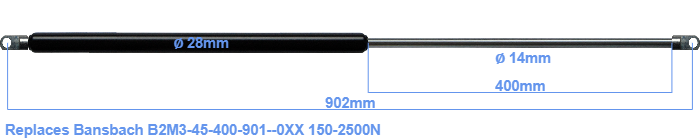# Replacement for Bansbach B2M3-45-400-901–0XX 150-2500N

\$60.51

Replacement gas spring for the Bansbach B2M3-45-400-901--0XX 150-2500 Newton. The eye has a thickness of 10mm and a hole diameter of 10.1mm. Brand: HAHN, a Stabilus company.

Please note:
• Mounting part tube: The thickness of the eye of this replacement is 10mm instead of 8mm.
• Mounting part rod : The thickness of the eye of this replacement is 10mm instead of 18mm.
•  Force Choose an option150 Newton200 Newton250 Newton300 Newton350 Newton400 Newton450 Newton500 Newton550 Newton600 Newton650 Newton700 Newton750 Newton800 Newton850 Newton900 Newton950 Newton1000 Newton1050 Newton1100 Newton1150 Newton1200 Newton1250 Newton1300 Newton1350 Newton1400 Newton1450 Newton1500 Newton1550 Newton1600 Newton1650 Newton1700 Newton1750 Newton1800 Newton1850 Newton1900 Newton1950 Newton2000 Newton2050 Newton2100 Newton2150 Newton2200 Newton2250 Newton2300 Newton2350 Newton2400 Newton2450 Newton2500 NewtonClearThe diameter of the black cylinder of this gas spring is 28 millimeter. The diameter of the rod is 14 millimeter. The rod (the chrome part) is 400 mm long. In total, the length is 902 millimeter. This is the total distance between the centers of the two mounting parts. The distance from thread to thread (without mounting parts) is 848 mm. This replacement gas spring has a force of 150-2500 Newton. This gas spring can replace a Bansbach gas spring, but is not an orignal Bansbach one. This is a HAHN gas spring. The force (150-2500 N) and dimensions are almost entirely identical to the original.